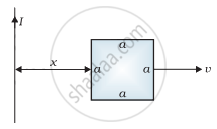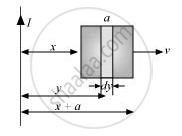# (a) Obtain an expression for the mutual inductance between a long straight wire and a square loop of side an as shown in the figure. (b) Now assume that the straight wire carries a current of 50 A - Physics

Numerical

(a) Obtain an expression for the mutual inductance between a long straight wire and a square loop of side an as shown in the figure.

(b) Now assume that the straight wire carries a current of 50 A and the loop is moved to the right with a constant velocity, v = 10 m/s.

Calculate the induced emf in the loop at the instant when x = 0.2 m.

Take a = 0.1 m and assume that the loop has a large resistance.#### Solution

(a) Take a small element dy in the loop at a distance y from the long straight wire (as shown in the given figure).Magnetic flux associated with elementdy, "d"phi = BdA

Where,

dA = Area of element dy = a dy

B = Magnetic field at distance y

= (mu_0"I")/(2pi"y")

I = Current in the wire

mu_0 = Permeability of free space = 4π × 10−7 T m A−1

∴ "d"phi = (mu_0"Ia")/(2pi)("dy")/"y"

phi = (mu_0"Ia")/(2pi)int("dy")/"y"

y tends from x to a + x

∴ phi = (mu_0"Ia")/(2pi) int_"x"^("a" + "x")("dy")/"y"

= (mu_0"Ia")/(2pi) [log_"e""y"]_"x"^("a" + "x")

= (mu_0"Ia")/(2pi) log_"e"(("a" + "x")/"x")

for mutual inductunce M, the flux is given as :

phi = "MI"

∴ MI = (mu_0"Ia")/(2pi) log_"e"("a"/"x" + 1)

M = (mu_0"a")/(2pi) log_"e"("a"/"x" + 1)

(b) Emf induced in the loop, e = B’av = ((mu_0"I")/(2pi"x"))"av"

Given,

I = 50 A

x = 0.2 m

a = 0.1 m

v = 10 m/s

"e" = (4pi xx 10^-7 xx 50 xx 0.1 xx 10)/(2pi xx 0.2)

"e" = 5 xx 10^-5 V

Concept: Induced e.m.f. and Induced Current
Is there an error in this question or solution?

#### APPEARS IN

NCERT Physics Part 1 and 2 Class 12
Chapter 6 Electromagnetic Induction
Exercise | Q 6.16 | Page 232
NCERT Class 12 Physics Textbook
Chapter 6 Electromagnetic Induction
Exercise | Q 16 | Page 232

Share